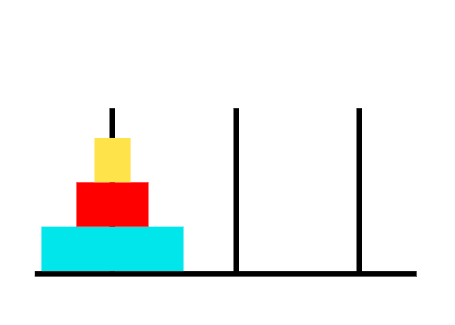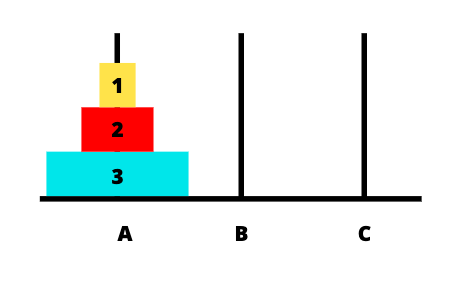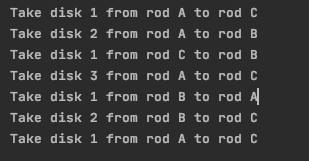# Tower of Hanoi - Algorithm and Implementation in Java

Published on August 3, 2022By Jayant Verma
Developer and author at DigitalOcean.While we believe that this content benefits our community, we have not yet thoroughly reviewed it. If you have any suggestions for improvements, please let us know by clicking the “report an issue“ button at the bottom of the tutorial.

The Tower of Hanoi is a classic problem in the world of programming. The problem setup consists of three rods/pegs and n disks.

The disks can be moved from one peg to another. The n disks are of different sizes.

Initially all the disks are stacked on the first tower. The disks are stacked in such a way that a disk is always over a disk bigger than itself.

## Tower of Hanoi Default SetupFun fact : This game was invented by a French mathematician Édouard Lucas in the 19th century. It is associated with a legend of a Hindu temple where the puzzle was supposedly used to increase the mental discipline of young priests.

### Problem Statement

``````Move all the disks stacked on the first tower over to the last tower using a helper tower in the middle. While moving the disks, certain rules must be followed. These are :

1. Only one disk can be moved.

2. A larger disk can not be placed on a smaller disk.
``````

So you need to move all the disks from the first tower over to the last. You can only move one disk at a time and never place a smaller disk over a larger disk.

To do this you have an extra tower, it is known as helper/auxiliary tower.

Since you can only move one disk at a time, the disk you move will have to be at the top of its tower.

### Theoretical Solution to the Tower of Hanoi Problem

Let’s name the towers as A,B,C and the disks as 1,2,3.We solve this question using simple recursion. To get the three disks over to the final tower you need to :

1. Take the disk number 1 and 2 to tower B.
2. Move disk number 3 to tower C.
3. Take disk number 1 and 2 from B to C.

Of course, you can’t do it like this because of the constraints. However, we can use this to create a function that does it recursively.

In the function we write, we will print the movement of the disks.

## Implementing the Solution to Tower of Hanoi in Java

Let’s begin with understanding the two main parts of the code to solve the Tower of Hanoi problem.

### 1. Base case

The base case in our code is when we only have one disk. That is n=1.

`````` if (n == 1)
{
System.out.println("Take disk 1 from rod " +  from_rod + " to rod " + to_rod);
return;
}
``````

### 2. Recursive calls

The recursive calls to solve tower of Hanoi are as follows:

`````` towerOfHanoi(n-1, from_rod, helper_rod, to_rod);
System.out.println("Take disk " + n + " from rod " +  from_rod + " to rod " + to_rod);
towerOfHanoi(n-1, helper_rod, to_rod, from_rod);
}
``````

These are equivalent to:

1. Move the top n-1 disks to the auxiliary tower.
2. Move 1 disk from source rod to destination rod.
3. Take the n-1 disks from auxiliary disk to the destination rod.

### Complete Java Implementation of Tower of Hanoi

``````package com.JournalDev;
public class Main {
static void towerOfHanoi(int n, char from_rod, char to_rod, char helper_rod)
{
if (n == 1)
{
System.out.println("Take disk 1 from rod " +  from_rod + " to rod " + to_rod);
return;
}
towerOfHanoi(n-1, from_rod, helper_rod, to_rod);
System.out.println("Take disk " + n + " from rod " +  from_rod + " to rod " + to_rod);
towerOfHanoi(n-1, helper_rod, to_rod, from_rod);
}

public static void main(String args[])
{
int n = 5;
towerOfHanoi(n,'A','C', 'B');
}

}

``````

The output for the code is:

``````Take disk 1 from rod A to rod C
Take disk 2 from rod A to rod B
Take disk 1 from rod C to rod B
Take disk 3 from rod A to rod C
Take disk 1 from rod B to rod A
Take disk 2 from rod B to rod C
Take disk 1 from rod A to rod C
``````We can understand the process using the following illustration. You can run the code for any number of disks.

The output for n=5 is :

``````Take disk 1 from rod A to rod C
Take disk 2 from rod A to rod B
Take disk 1 from rod C to rod B
Take disk 3 from rod A to rod C
Take disk 1 from rod B to rod A
Take disk 2 from rod B to rod C
Take disk 1 from rod A to rod C
Take disk 4 from rod A to rod B
Take disk 1 from rod C to rod B
Take disk 2 from rod C to rod A
Take disk 1 from rod B to rod A
Take disk 3 from rod C to rod B
Take disk 1 from rod A to rod C
Take disk 2 from rod A to rod B
Take disk 1 from rod C to rod B
Take disk 5 from rod A to rod C
Take disk 1 from rod B to rod A
Take disk 2 from rod B to rod C
Take disk 1 from rod A to rod C
Take disk 3 from rod B to rod A
Take disk 1 from rod C to rod B
Take disk 2 from rod C to rod A
Take disk 1 from rod B to rod A
Take disk 4 from rod B to rod C
Take disk 1 from rod A to rod C
Take disk 2 from rod A to rod B
Take disk 1 from rod C to rod B
Take disk 3 from rod A to rod C
Take disk 1 from rod B to rod A
Take disk 2 from rod B to rod C
Take disk 1 from rod A to rod C
``````

The formula to calculate the number of steps for n disks is :

``````(2^n)-1
``````

## Conclusion

This is how you solve the Tower of Hanoi using recursion. Hope you had fun learning with us!Developer and author at DigitalOcean.

#### Still looking for an answer?

How can this be extended for t number of towers, everyone i have seen targets three towers, what about 4, 5, 6, 7… towers?

- Oluwatosin